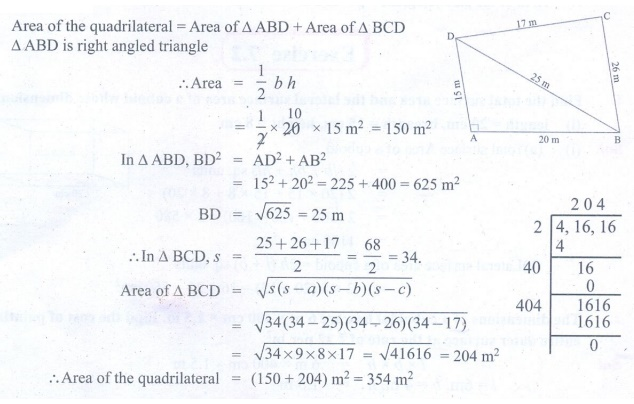Home | | Maths 9th std | Exercise 7.1: Application of Heronâ€™s Formula in Finding Areas of Quadrilaterals

# Exercise 7.1: Application of Heronâ€™s Formula in Finding Areas of Quadrilaterals

Maths : Mensuration: Book Back, Exercise, Example Numerical Question with Answers, Solution: Exercise 7.1: Application of Heronâ€™s Formula in Finding Areas of Quadrilaterals

Application of Heronâ€™s Formula in Finding Areas of Quadrilaterals

Exercise 7.1

1. Using Heronâ€™s formula, find the area of a triangle whose sides are

(i) 10 cm, 24 cm, 26 cm (ii) 1.8m, 8 m, 8.2 m2. The sides of the triangular ground are 22 m, 120 m and 122 m. Find the area and cost of levelling the ground at the rate of â‚ą 20 per m2.3. The perimeter of a triangular plot is 600 m. If the sides are in the ratio 5:12:13, then find the area of the plot.4. Find the area of an equilateral triangle whose perimeter is 180 cm.5. An advertisement board is in the form of an isosceles triangle with perimeter 36m and each of the equal sides are 13 m. Find the cost of painting it at â‚ą 17.50 per square metre.6. Find the area of the unshaded region.7. Find the area of a quadrilateral ABCD whose sides are AB = 13cm, BC = 12cm, CD = 9cm, AD = 14 cm and diagonal BD = 15cm.8. A park is in the shape of a quadrilateral. The sides of the park are 15 m, 20 m, 26 m and 17 m and the angle between the first two sides is a right angle. Find the area of the park.9. A land is in the shape of rhombus. The perimeter of the land is 160 m and one of the diagonal is 48 m. Find the area of the land.10. The adjacent sides of a parallelogram measures 34 m, 20 m and the measure of one of the diagonal is 42 m. Find the area of parallelogram.Tags : Numerical Problems with Answers, Solution | Mensuration | Maths , 9th Maths : UNIT 7 : Mensuration
Study Material, Lecturing Notes, Assignment, Reference, Wiki description explanation, brief detail
9th Maths : UNIT 7 : Mensuration : Exercise 7.1: Application of Heronâ€™s Formula in Finding Areas of Quadrilaterals | Numerical Problems with Answers, Solution | Mensuration | Maths